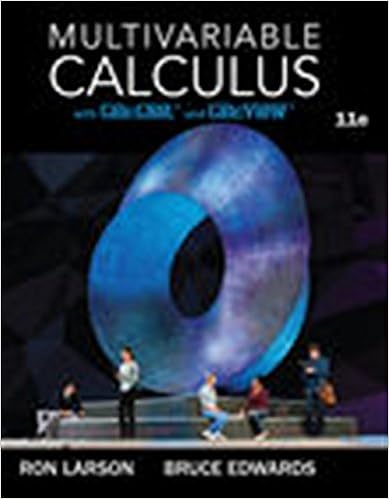# Section5.1.pdf - Math 140 Fall 2016 5.1 Areas between...

• Ammarnorain
• 2

This preview shows page 1 - 2 out of 2 pages.

##### We have textbook solutions for you!
The document you are viewing contains questions related to this textbook.The document you are viewing contains questions related to this textbook.
Chapter 11 / Exercise 67
Multivariable Calculus
LarsonExpert Verified
Math 140 Fall 2016§5.1 Areas between CurvesSuppose thatfis a continuous function on [a, b].1) Iff(x)0 forx[a, b], thenZbaf(x)dx=the area of the region that is below thegraph off, above thex-axis and between the linesx=aandx=b.2) Iff(x)0 forx[a, b], thenZbaf(x)dx=-the area of the region that is be-low the graph off, above thex-axis and between the linesx=aandx=b.ThusZba|f(x)|dx= the area of the region bounded by the graph off, thex-axis and the linesx=aandx=b.1234x12y12x123y0.20.40.60.81.01.21.47.07.58.08.59.0Supposefandgare continuous curves on [a, b]. We want to find the area enclosed by twocurvesy=f(x) andy=g(x) and the linesx=aandx=b.(1)The areaAof the region bounded by the graphs of two continuos curvesy=f(x) andy=g(x), and the linesx=a, andx=b, wheref(x)g(x) for allxin [a, b], is defined asA=Zba[f(x)-g(x)]dx=Zba[yT-yB]dxwhereyTis the top curve, andyBis the bottom curve.(2)The areaAof the region bounded by the curvex=f(y), x=g(y), and the linesy=candy=d, wheref(y)g
##### We have textbook solutions for you!
The document you are viewing contains questions related to this textbook.The document you are viewing contains questions related to this textbook.
Chapter 11 / Exercise 67
Multivariable Calculus
LarsonExpert Verified
•••